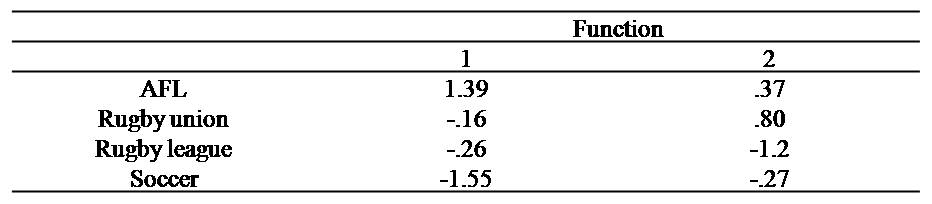# Discriminant function analysis

### Introduction

Like MANOVAs, Discriminant Function analysis is used to compare groups, like the two sexes, on more than one numerical variable at the same time, such as IQ and wage. Nevertheless, discriminant function analysis can provide more information, but is usually applied only when you want to examine one independent variable at a time. This article does assume some knowlege of MANOVA.

To illustrate discriminant function analysis, consider a researcher who wants to determine whether or not the values and objectives of footballers vary across the four codes: AFL, rugby league, rugby union, and soccer. Each individual rates the extent to which they value the money, influence, enjoyment, variety, mateship, and networking that emerges from football. An extract of the data is presented below. In this instance, 1 denotes AFL, 2 denotes league, 3 denotes union, and 4 denotes soccer.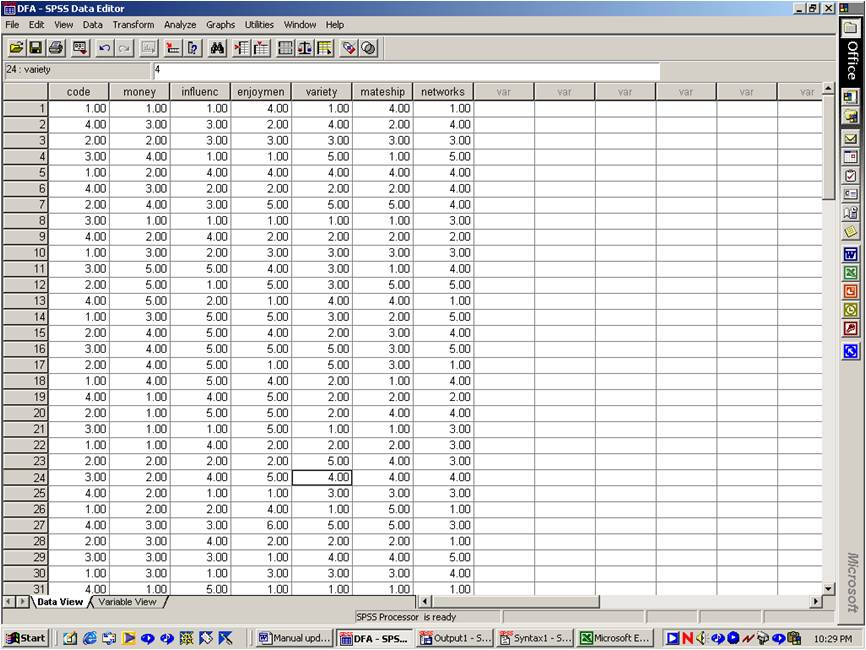To determine whether or not the four football codes differ from one another, the researcher could undertake a series of ANOVAs. Each ANOVA could apply to a separate value. For example, the first ANOVA could ascertain whether or not money varies across the four codes.

This approach, however, yields two principal drawbacks. The first problem relates to the family-wise Type I error rate. Nevertheless, many procedures could be utilised to circumvent this problem, such as Bonferroni adjustments, MANOVAs, and so forth.

The second problem relates to the interpretation of these outcomes. The table below presents the mean score on every value for each code. Even if all these values differed significantly from one another, interpretations would be clouded. One value is emphasized by AFL players more than other footballers. The next value is emphasized by Rugby League players more than other footballers, and so forth. The pattern that emerges is intricate. Clear, informative interpretations and implications are difficult to reach. Discriminant function analysis is intended to summarise this pattern to derive simple, informative conclusions.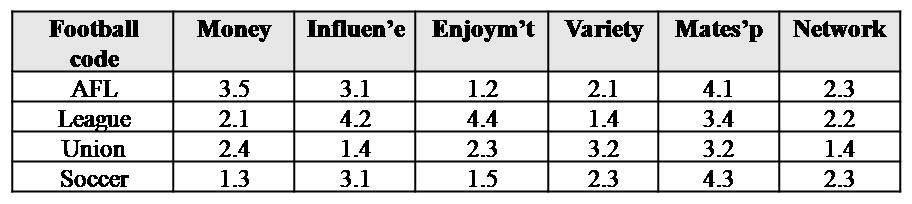### Step 1: Implement the analysis

To conduct a discriminant function analysis:

1. Select "Classify" and then "Discriminant" from the "Analyse" menu, after opening your data file.
2. Specify the grouping variable--such as "code"--in the appropriate box.
3. Press "Define range" and specify the highest and lowest values of the grouping variable in the corresponding spaces before pressing "Continue".
4. Specify the measures, such as Money, Influence, and so forth, in the box labelled "Independents".
5. As an option, select the "Statistics" button and tick the option adjacent to Fishers.
6. Press Paste. This button copies the instructions that pertain to your selections into a syntax file. Indeed, some researchers always press "Paste" rather than "OK" to retain a record of their analyses.
7. In the second last row, type "/ROTATE =structure". Do not include the quotation marks, however.
8. Execute this syntax by highlighting the instructions using the mouse and then choosing "Selection" from the "Run" menu.

### Step 2: Number of significant dimensions

The table below presents the first set of output that needs to be explored. In particular, the researcher merely needs to determine the number of significant p values in the final column. In this instance, 2 of the p values are less than 0.05 and thus significant. This value indicates the football codes vary on 2 distinct facets or dimensions.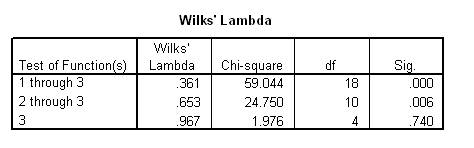For example, perhaps the football codes differ according to the extent to which they value extrinsic rewards and intrinsic satisfaction but not social interactions. One the other hand, perhaps the football codes differ according to the extent to which they value short and medium term values but not future goals. Unfortunately, this table does not delineate the facets or dimensions that differentiate the football codes. Instead, this information merely indicates that football codes vary along two facets or dimensions.

To delineate or interpret these facets or dimensions, the structure matrix needs to be explored. The following subsections first present the rationale that underpins this matrix. Another section will delineate the process that needs to be conducted to utilise this matrix.

### Rationale behind discriminant function analysis

To appreciate the significance of a structure matrix, the rationale that underlies a discriminant function analysis needs to be understood. In particular, discriminant function analysis combines the various measures into a single column, which is variously called a function, dimension, variate, or linear combination. For example, SPSS might invoke the following formula to create this new column

Function 1 = 2.5 x Money + 3 x Influence + 1.5 x Enjoyment + 4 x Variety + 2 x Mateship + 3.5 x Networks

The table below displays an extract of the data, together with the new column. The following table presents the average score of this function for each football code. In practice, this function does not appear in the SPSS datasheet, but is presented here to facilitate learning.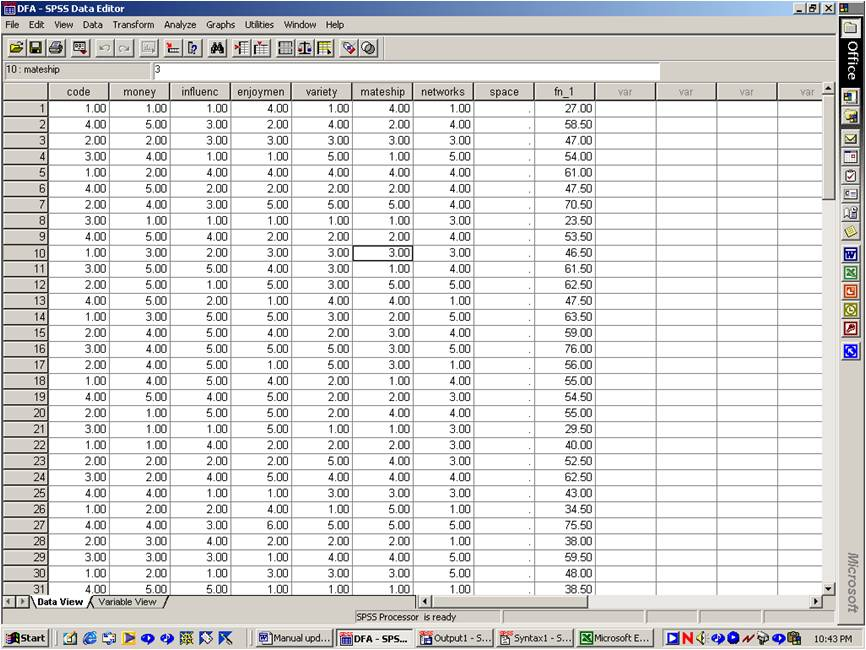#### Average of Function 1 in each group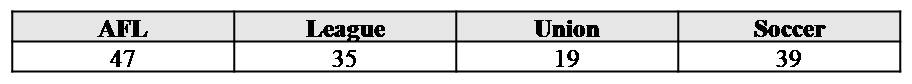Of course, SPSS does not utilise the same formula on each occasion. Instead, SPSS selects the coefficients--the numbers that precede each variable--carefully. In particular, SPSS selects the coefficients that maximize the difference between the groups. In other words, roughly speaking, any other formula would have generated averages that varied across the football codes to a lesser extent. Strictly speaking, any other formula would have generated a higher Wilks Lambda--which is the variability of these scores within groups divided by the total variability of these scores (see MANOVAs).

### Multiple dimensions

Unfortunately, a single function or new column is not sufficient: This column alone does not capture the entire pattern of findings. To illustrate, the previous table reveals that AFL footballers yield higher scores than other codes. In reality, however, soccer players may yield higher scores on some values, Rugby League players may yield higher scores on some other values, and so forth. Several functions should thus be created to capture this intricate pattern. Indeed, SPSS computes several functions or additional columns.

Specifically, the number of functions that SPSS calculates equals the number of groups minus 1 or the number of numerical variables, whichever is smaller. In this instance, the number of groups-1 equals 3. The number of numerical variables equals 6. Hence, SPSS will compute 3 functions in this context. These three functions or new columns are presented in the following table. The mean of each function is 0 and the standard deviation is 1.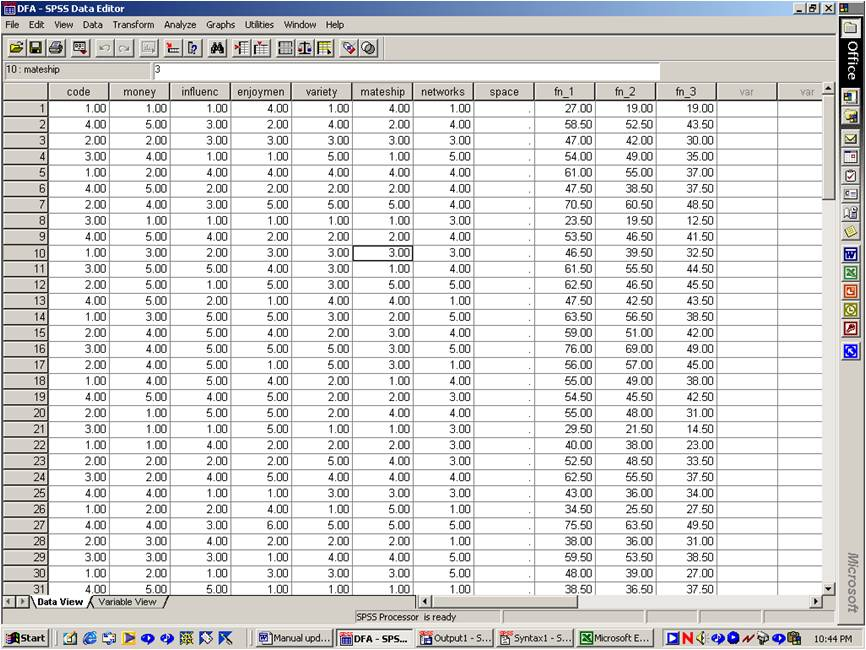For example, to construct the second new column, SPSS might have utilised the following formula:

Function 2 = 1.5 x Money + 2 x Influence + 4.5 x Enjoyment + 0.5 x Variety + 6 x Mateship + 4 x Networks

In particular:

• SPSS again ascertains a formula that is intended to maximise the difference between these groups with the condition the second function must be uncorrelated with the first function. /li>
• That is, high scores on the second column must not necessarily coincide with high scores on the first column.
• If this restriction was not instituted, these two columns would be almost identical and thus redundant.
• A similar principle applies to the other functions or additional columns. For example, the third function is uncorrelated with the first and second functions, and so forth.

### Step 3: Interpret the significant functions

Consider the previous table again. Theoretically, you could compute the correlation between each measure and each function. For example, you could compute the correlation between the Money scores and Function 1 scores. A high correlation would obviously suggest that increases in Money responses tend to coincide with increases in Function 1 scores. These correlations, therefore, provide information that researchers can use to define or understand the functions. Indeed, SPSS actually presents the correlations between all the measures and all the functions. The table that presents these correlations is usually called a "structure matrix". A typical structure matrix is provided below.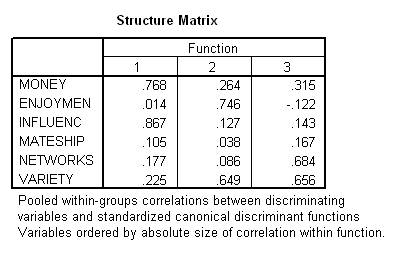This structure matrix or table can be utilised to interpret the functions or additional columns. In particular, higher correlations, such as values that exceed 0.4 or so, obviously reflect measures that pertain to the corresponding function.

To illustrate, consider a researcher who needs to interpret the first function.

• Clearly, only Money and Influence seem to correlate with this function.
• Accordingly, the first function seems to reflect Money and Influence but not the other values.
• One reasonable interpretation would be that Money and Influence seem to reflect extrinsic incentives.
• Hence, Function 1 might represent extrinsic values.
• Given that Function 1 achieved significance, we can thus conclude the extent to which footballers value extrinsic incentives varies across the football codes.

Consider the second function. In this instance,

• Only Enjoyment and Variety seem to correlate with this function.
• Accordingly, the second function seems to reflect intrinsic attributes of the task.
• Given that Function 2 achieved significance, we can thus conclude the extent to which footballers value intrinsic attributes varies across the football codes.

Furthermore, we do not need to interpret the third function, because this variate did not differ significantly across the football codes. That is, only two of the functions achieved significance. By definition, then, the third function could not be significant.

In most instances, however, the functions cannot be interpreted this readily. Typically, most of the values in this structure matrix are moderate. Hence, the functions cannot be distinguished easily. To overcome this obstacle, researchers can examine the rotated structure matrix instead. This matrix ensures the numbers are either high or low, rather than moderate, which facilitates interpretations.

### Step 4: Assess stability

Sometimes, the results that emerge from Discriminant Function Analyses are unstable. That is, minor adjustments to the responses could have generated vastly different outcomes. Several techniques can be undertaken to assess the stability of these outcomes, such as cross-validation. Perhaps the most straightforward technique is to examine the standardized canonical coefficients, as depicted below. In particular, the sign of these coefficients should match the sign of values displayed in the structure matrix. If, for example, a negative value in this table corresponds to a positive value in the structure matrix, the outcomes may be unstable. Note, however, that SPSS varies the order of variables. For example: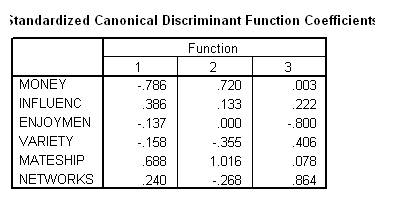• In the previous structure matrix, the final row pertained to "variety".
• In the standardised canonical matrix, the final row pertained to "networks".

As an aside, to understand the source of these coefficients, recall that several formulas were used to create the functions or additional columns, such as:

Function 1 = 2.5 x Money + 3 x Influence + 1.5 x Enjoyment + 4 x Variety + 2 x Mateship + 3.5 x Networks

Function 2 = 1.5 x Money + 2 x Influence + 4.5 x Enjoyment + 0.5 x Variety + 6 x Mateship + 4 x Networks

Function 3 = 1 x Money + 1.5 x Influence + 3 x Enjoyment + 2 x Variety + 3.5 x Mateship + 2 x Networks

Roughly speaking, the standardized coefficients are the values in these formulas. Strictly speaking, the standardized coefficients are the values in the formulas that would have been utilised had the data first been converted to z scores.

### Step 5: Comparison of groups

The results thus far merely indicate that extrinsic and intrinsic values vary across the football codes. These results do not indicate which football codes emphasize extrinsic and intrinsic values to the least and greatest extent. The following table, together with the structure matrix, needs to be scrutinized to resolve this question.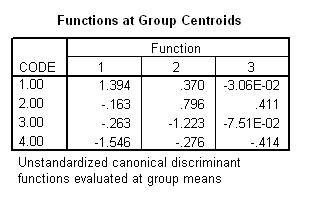This table reports the average score of every function for each group separately. To illustrate, consider the first function only. In this instance, AFL footballers generated the highest average score on this function and soccer players generated the lowest average score. In addition, the structure matrix revealed that high scores on the first function coincide with high scores on extrinsic values. Accordingly, these findings, taken together, indicate that footballers emphasize extrinsic values to a greater extent than do Rugby players who emphasize extrinsic values to a greater degree than do soccer players. The same process can be applied to the second function.

### Step 6: Classification of other individuals

Discriminant function analysis can also provide many other benefits. For example, you can create equations that enable you to predict the group membership of individuals from the measures alone. As an illustration, you could predict whether an individual, who has yet to decide which sport they prefer, is more likely to play AFL than another code from their values. In particular, SPSS can generate the following table.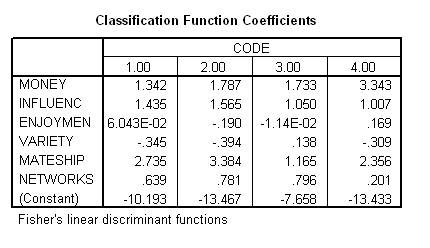This table provides coefficients that can be utilised to generate a set of equations. Specifically, using these coefficients, the following equations emerge.

AFL = 1.342 x Money + 1.435 x Influence + 0.060 x Enjoyment -0.345 x Variety + 2.735 x Mateship + 0.639 x Networks - 10.193

League = 1.787 x Money + 1.565 x Influence + -0.19 x Enjoyment + -0.394 x Variety + 3.384 x Mateship + 0.781 x Networks - 13.467

Union = 1.733 x Money + 1.050 x Influence - 0.0114 x Enjoyment + 0.138 x Variety + 1.165 x Mateship + 0.796 x Networks - 7.658

Soccer= 3.343 x Money + 1.007 x Influence + 0.169 x Enjoyment - 0.309 x Variety + 2.356 x Mateship + 0.201 x Networks - 13.433

To predict the group classification from the measures alone:

• Substitute the responses of some individual on each value into these formulas.
• For example, suppose some individual specified a value of 3 for Money, 4 for Influence, 2 for Enjoyment, 5 for Variety, 2 for Mateship, and 3 for Networks.
• These values would then be substituted into each of the four formulas.
• The formula that yields the highest score corresponds to the group to which this individual is most likely to belong.

Before undertaking discriminant function analysis for this purpose, you should press "Classify". Select the option "compute from group sizes" only if the proportion of sampled individuals that pertain to each group represents the proportion of individuals in the population that pertain to each group. Otherwise, retain the default "all groups equal". To illustrate:

• Suppose that 50% of the sample play AFL, 20% play League, 20% play Union, and 10% play soccer.
• Suppose that similar percentages of the Australian population play each sport.
• Hence, the researcher would prefer SPSS to bias the classification equations to reflect these percentages.
• That is, the researcher would prefer the equations to ensure that 50% of new individuals are likely to be classified as AFL players, 20% as League players, and so forth.
• The option "compute from group sizes" ensures the equations are biased appropriately.

Suppose instead that 50% of the sample play AFL, 20% play League, 20% play Union, and 10% play soccer. Suppose these percentages do not reflect the popularity of each sport in the Australian population. Hence, the researcher would prefer SPSS not to bias the classification equations to reflect the percentage of each sport in the sample. That is, the researcher would instead prefer the equations to ensure that 25% of new individuals are likely to be classified in each sport. The option "all groups equals" ensures the equations are unbiased.

### Illustration of the format used to report discriminant function analysis

A discriminant function analysis was conducted to uncover the dimensions of values that differentiate four football codes: AFL, soccer, rugby union, and rugby league. The values included the extent to which the individuals perceive money, influence, enjoyment, variety, mateship, and networking as important to their lives. The first and second functions significantly differentiated the groups, Wilks Lambda= .361, Chi square (18) = 59.4, p < 0.001, and Wilks Lambda= .653, Chi square (10) = 24.75, p < 0.01 respectively. The third function did not reach significance.

The structure matrix and group centroids for the first and second function are presented in Tables 1 and 2 respectively. According to the structure matrix, the first function primarily represents money and influence, which can be conceptualised as extrinsic drives. The group centroids suggest this function, and thus extrinsic drives, tend to be most elevated in AFL players and least pronounced in soccer players.

Finally, the second function seems to represent enjoyment and variety, which can be conceptualised as intrinsic motivation. The group centroids indicate that intrinsic motivation seems to be highest in rugby union and lowest in rugby league players.

Table 1. Structure matrix that emerged from the discriminant function analysis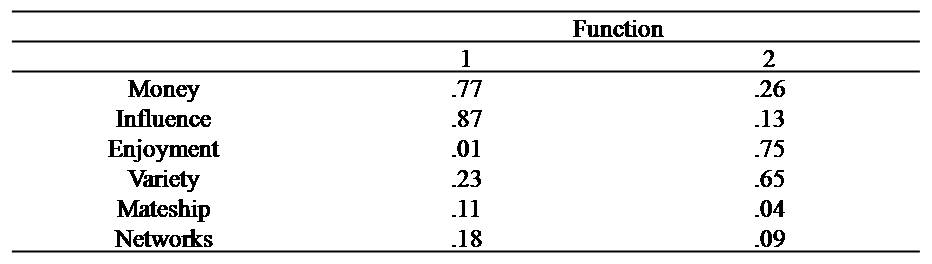Table 2. Group centroids that emerged from the discriminant function analysis